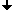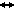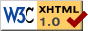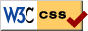﻿ Seminar: Shock waves, Winter 2015/16
Nonlinear Dynamics at the Free University Berlin
Winter 2015/16

# Seminar: Shock waves

## Schedule, Winter 2015/16

Seminar:
Monday 10.00-12.00, SR 140 / Arnimallee 7

## Description

We will study shock waves (i.e. a disturbance skirting regions with tremendously different properties e.g. of density, pressure, temperature) arising in systems of 1st order PDEs modeling e.g. transport phenomena, conservation laws, gas dynamics, wave equations, traffic flows. On this way, we will learn main notions and tools for the analysis of 1st order PDEs, in particular, characteristics, weak solutions, entropy, viscosity and asymptotics of solutions.

The language of the seminar is supposed to be English (with the help of German if needed).

Interested students are supposed to be acquainted with ordinary differential equations and analysis and should furthermore possess basic knowledge about partial differential equations.

## Topics

### Method of characteristics

1. Talk: Introduction
What are non-linear first order PDEs. Examples. Derivation of the characteristic ODE system.
2. Talk: Local solution theory
Non-characteristic surface condition. Existence and uniqueness of local solution via characteristic ODE system. Example: Quasilinear equations.

### Scalar Conservation Laws

3. Talk: Examples of main phenomena
What are conservation laws? How do they appear? Examples: Gas dynamics, Burgers equation. Canonical pictures of singularities. Rankine-Hugoniot conditions. Entropy condition. Weak solutions.
4. Talk: Hopf-Lax formula I
Solution of the variational problem. Theorem 4 in Evans Section 3.3.2
5. Talk: Hopf-Lax formula II
Euler-Lagrange equation and nonlinear first order systems. Theorem 5/6 Evans Section 3.3.2. Example: See Evans Section 3.3.3 with appropriate initial condition
6. Talk: Uniqueness of weak solutions
Uniqueness criteria. Theorem 7/8 Evans Section 3.3.3
7. Talk: Existence of weak solution of conservation laws
Lax-Oleinik formula. Theorem 1/2 Evans Section 3.4.2
8. Talk: Uniqueness of weak solutions
Entropy conditions revisited. Uniqueness of entropy solutions. See Evans Section 3.4.3
9. Talk: Asymptotic behaviour
Convergence in $L^\infty$ and in $L^1$. N-Waves.

### System of conservation laws

10. Talk: Introduction
Semilinear equations. Traveling waves. Hyperbolicity. Example: Euler equations. Weak solution of the Riemann problem. Rarefaction and shocks.
11. Talk: Singularities in systems I
Simple waves and rarefaction waves in the Riemann problem.
12. + 13. Talks: Shocks in systems I & II
Linear degenerate shock waves. Shock set. Nonlinear setting. Evans 11.2.3
14. Talk: Solution of Riemann problem
Solution of Riemann problem by singularities. Evans 11.2.4.
15. Talk: Viscosity solutions and traveling waves
Viscosity solution. Traveling waves. Examples.

### Literature

• Lawrence C. Evans. Partial differential equation.
• Joel Smoller. Shock waves and Reaction-Diffusion Equations.
• Constantine M. DaFermos. Hyperbolic conservation laws in continuum physics.Last change: Oct. 6, 2015
 This page strictly conforms to the XHTML1.0 standard and uses style sheets.